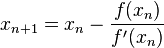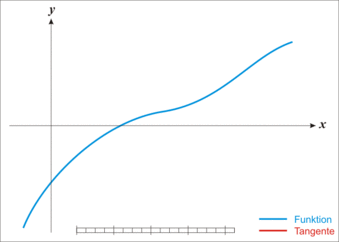# Newton's method facts for kids

Kids Encyclopedia Facts

Newton's method provides a way for finding the real zeros of a function. This algorithm is sometimes called the Newton–Raphson method, named after Sir Isaac Newton and Joseph Raphson.

The method uses the derivative of the function in order to find its roots. An initial "guess value" for the location of the zero must be made. From this value, a new guess is calculated by this formula:$x_{n+1} = x_n - \frac{f(x_n)}{f'(x_n)}$

Here xn is the initial guess and xn+1 is the next guess. The function f (whose zero is being solved for) has the derivative f'.

By repeatedly applying this formula to the generated guesses (that is by setting the value of xn to the formula's output and recomputing), the value of the guesses will approach a zero of the function.The function (blue) is being used to calculate the slope of a tangent line (red) at xn.

Newton's method can be explained graphically by looking at intersections of tangent lines with the x-axis. First, a line tangent to the f at xn is calculated. Next, the intersection between this tangent line and the x-axis is found. Finally, the x-position of this intersection is recorded as the next guess, xn+1.

## Problems with Newton's Method

Newton's method can find a solution quickly if the guess value begins sufficiently near the desired root. However, when the initial guess value is not close, and depending on the function, Newton's method may find the answer slowly or not at all.Newton's method Facts for Kids. Kiddle Encyclopedia.